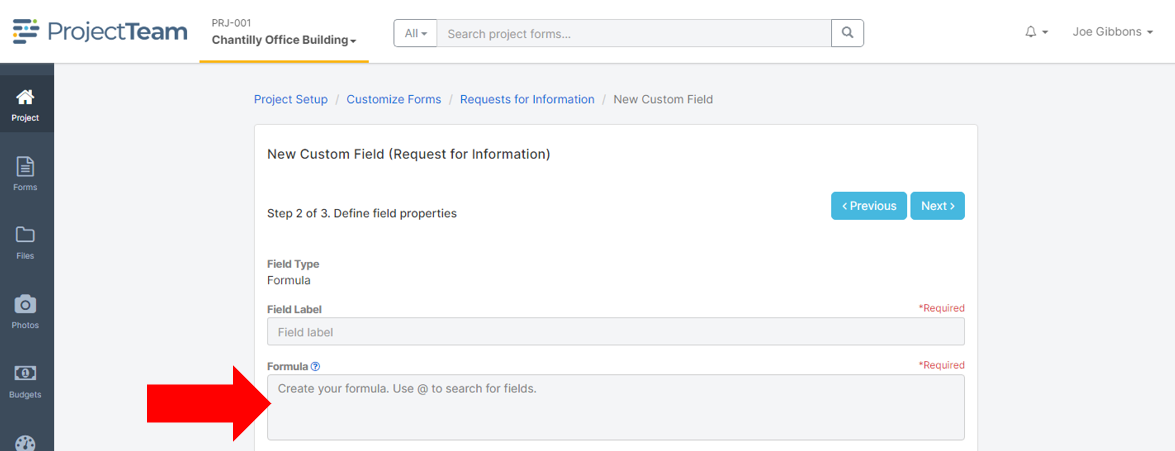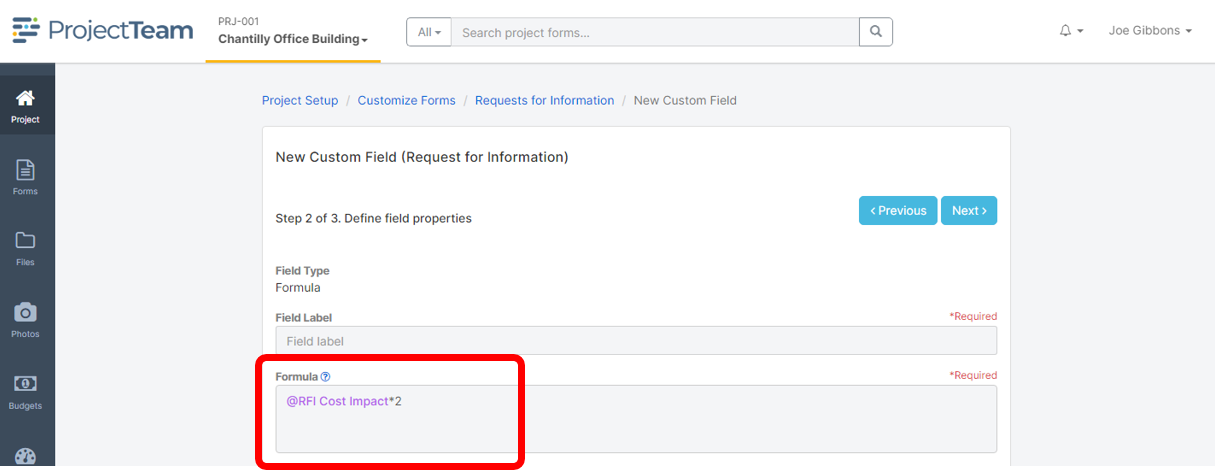Help Center Home

# Custom Formula fields

## This article walks through how to create a custom formula field.

### Overview

Formula fields are a powerful custom field type that can be used to manipulate the data added to your forms. Formula fields allow you to calculate data using both simple and advanced functions against constants and fields on your form. Custom formula fields can be added as Company Fields which can be added to many projects or as standalone custom fields on an individual project.

Example use cases where you might want a custom Formula field include:

• Calculate the difference between two dates (how many days are between the due date and today's date)
• Subtract a number from an input date (due date minus 14 days is when we need our materials)
• Multiply numbers together (length times width to get total area)

### Creating a formula

When adding a new custom Formula field, you will notice a box labeled "Formula". This box is where you type your custom formula.You can use constants and fields as variables in your Formula field. Use the "@" symbol to search for fields available on the form type. When you type "@" you will see a dropdown that lists fields that you have access to. Once a field is selected, it shows as purple text to help you clearly see which parts of your formulas are based on fields.### What field types are supported in formulas?

You can create formulas based on the following field types:

• Checkbox
• Collection
• Date
• Email
• Number
• Number (Currency)
• Phone
• Project Directory (Contact)
• Text (Multiline
• Text (Single line)
• URL

### What field types are not currently supported in formulas?

• Account Codes
• Reference Collection
• Cost Period
• Formula
• Number (Auto Number)
• Picklist
• Text (Multiline Rich Format)

### Simple Formulas

The Formula box supports simple addition (+), subtraction (-), multiplication (*), and division (/) operators for numerical calculations. Example functions using these simple operators include (don't forget about PEMDAS):

• 2+2
• @RFI Cost Impact*3.5
• (2*(@Number of Units+150))/4

We support many of the common functions used in your favorite spreadsheet applications which are broken down into five basic categories: Aggregate, Arithmetic, Date, Logic, and Strings.

#### Aggregate Functions

 Syntax Example Expected Result AVG(number1, {number2, ...}) Return average of numbers supplied. AVG(2, 4, 8, 16) 7.5 MAX(number1, {number2, ...}) Return the biggest value from the numbers supplied. MAX(2, 4, 8, 16) 16 MIN(number1, {number2, ...}) Return the smallest value from the numbers supplied. MIN(2, 4, 8, 16) 2 SUM(number1, {number2, ...}) Return sum of numbers supplied. SUM(2, 4, 8, 16) 30

#### Arithmetic Functions

 Syntax Example Expected Result ABS(number) Returns the absolute value of a given number. ABS(-2.6) 2.6 CEILING(number) Rounds a given number away from zero, to the nearest multiple of a given number. CEILING(2.6) 3 FLOOR(number) Rounds a given number towards zero to the nearest multiple of a specified significance. FLOOR(2.6) 2 ROUND(number, number_of_decimals) Rounds a number to a fixed number of decimal places. ROUND(2.345, 2) 2.35

#### Date Functions

 Syntax Example Expected Result DATEADD(number, date) Adds a number of days to a date. DATEADD(2, '8/15/2022') 8/17/2022 DATEDIFF(date1, date2) Calculates the number of days between two dates. DATEDIFF('8/15/2022','8/30/2022') 15 TODAY() Returns the current date in the current user’s time zone. TODAY() Today

#### Logical

 Syntax Example Expected Result AND(logical_expression1, [logical_expression2, {...}]) Determine if all conditions are TRUE. AND(true, false, true) False IF(logical_expression, value_if_true, value_if_false) Returns TRUE when a given string is null or empty, otherwise, return FALSE. IF(true, 'Yes', 'No') Yes ISBLANK() Returns TRUE when a given string is null or empty, otherwise, return FALSE. ISBLANK(Null) True NOT(logical_expression) To confirm one value is not equal to another. NOT(false) True OR(logical_expression1, [logical_expression2, {...}]) Determine if any conditions in a test are TRUE. OR(true, false, true) True SWITCH(expression, case1, value1, [default or case2, value2], {...}) Compares a list of values to a reference value and, of the first to equal the reference, returns its partner. If none are equal, returns null. SWITCH(4, 2, 'Two', 4, 'Four', 8, 'Eight', 'Other') Four

#### String

 Syntax Example Expected Result CONCAT(string1, {string2, ...}) Combines the text from multiple strings. CONCAT('This',' ', 'is', ' ', 'cool!') This is cool! INDEXOF(character, string) Returns the location of a substring in a string (case sensitive). INDEXOF('n', 'Construction') 3 LEFT(string, number) Extracts a given number of characters from the left side of a supplied text string. LEFT('Construction', 3) Con LEN(string) Gets the length (number of characters) of a string. LEN('Construction') 12 LOWER(string) Converts all letters in the specified string to lowercase. LOWER('Construction') construction MID(string, starting_number, number_characters) Extracts a given number of characters from the middle of a supplied text string. MID('Construction',4,9) struction REPLACE(string, string, string) Replaces characters of a string specified in a given text string with another text string. REPLACE('Do this', 'this', 'that') REPLACE('Replace all of the es', 'e', 'X') Do that RXplacX all of thX Xs REVERSE(string) Reverse a string. REVERSE('Racecar') racecaR RIGHT(string, number) Extracts a given number of characters from the right side of a supplied text string. RIGHT('Construction',3) ion TRIM(string) Removes extra spaces from text. TRIM('   Construction') Construction UPPER(string) Converts all letters in the specified string to uppercase. UPPER('Construction') CONSTRUCTION